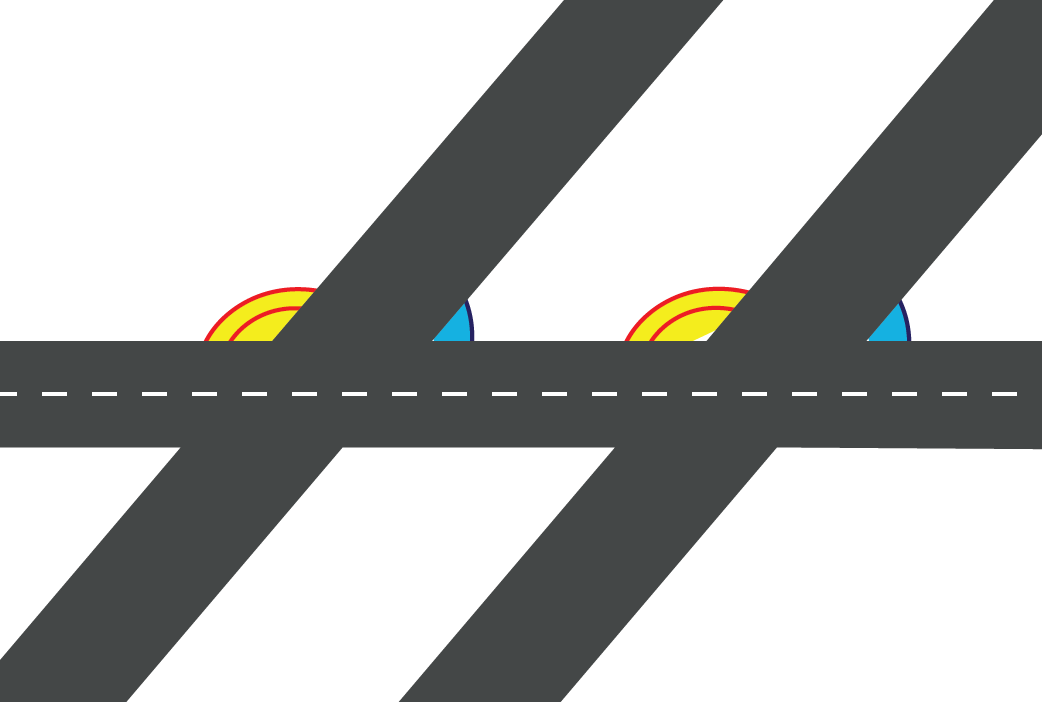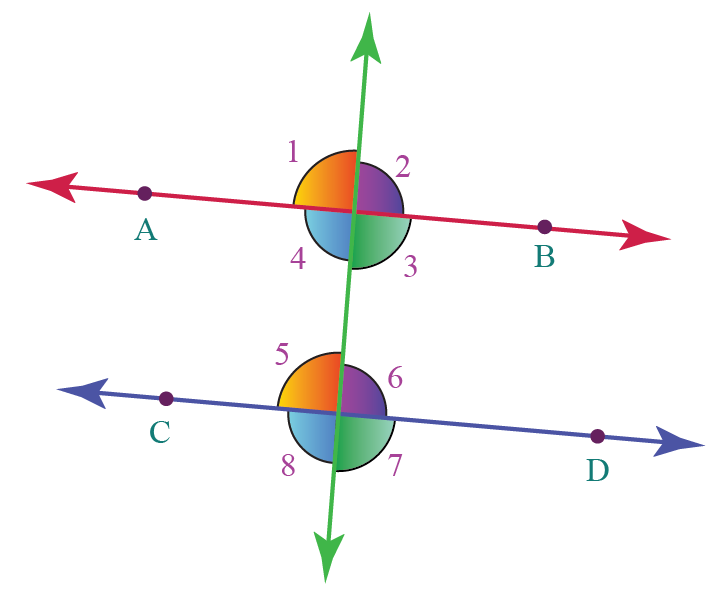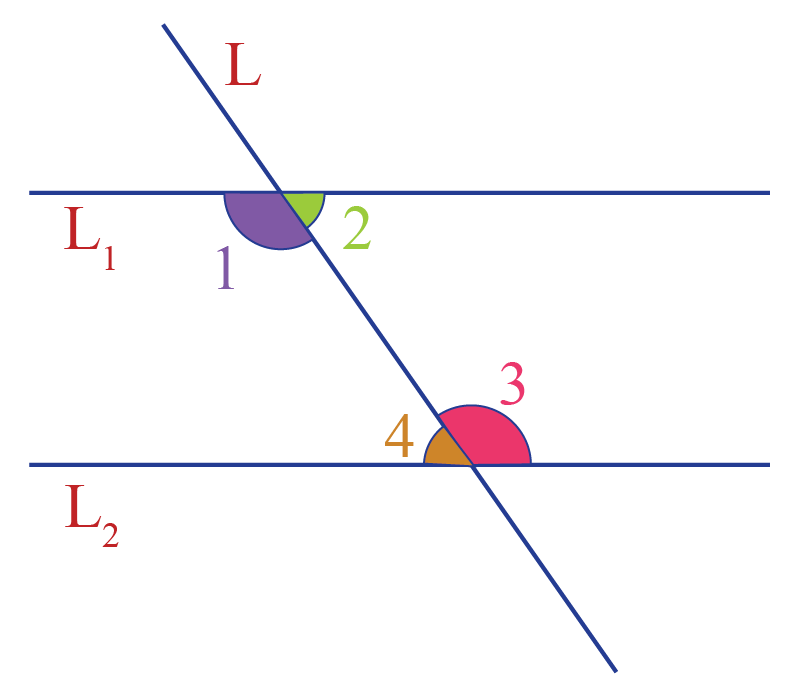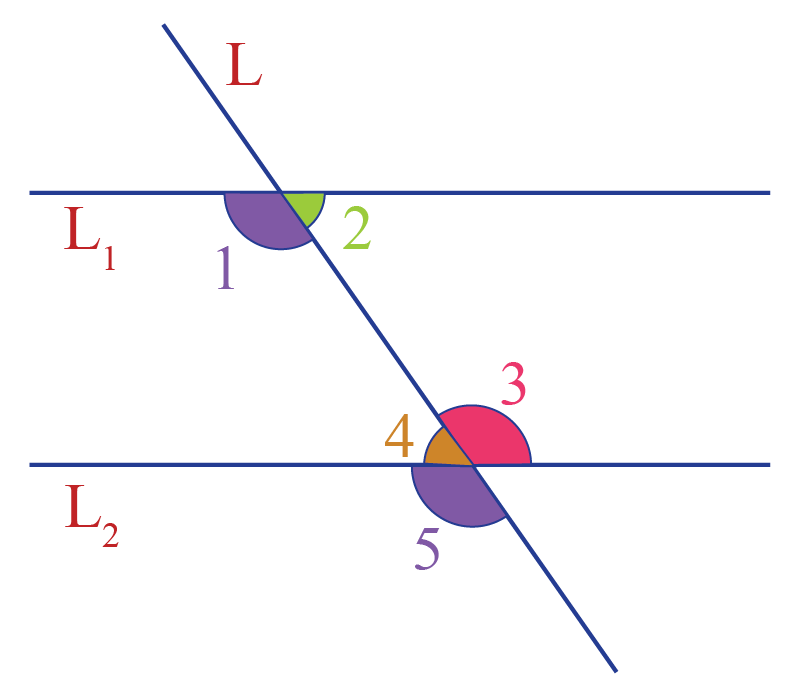# Same side interior angles

Same side interior angles

Interior angles are fun to play around with once you know what exactly they are, and how to calculate them. Understanding interesting properties like the same side interior angles theorem and alternate interior angles help a long way in making the subject easier to understand.

Here is what happened with Ujjwal the other day.

Ujjwal was going in a car with his dad for a basketball practice session. On the way to the ground, he saw many roads intersecting the main road at multiple angles.That's when his curiosity grew as to what is the relation between the angles created by the roads.

Let’s find out!

## Lesson Plan

 1 What Are Same Side Interior Angles? 2 Important Notes on Same Side Interior Angles 3 Solved Examples on Same Side Interior Angles 4 Challenging Questions on Same Side Interior Angles 5 Interactive Questions on Same Side Interior Angles

## What Are Same Side Interior Angles?

When two parallel lines are intersected by a transversal, 8 angles are formed.We will study more about "Same Side Interior Angles" here.

The same side interior angles are the pair of non-adjacent interior angles that lie on the same side of the transversal.

So the same side interior angles:

• have different vertices
• lie between two lines
• and are on the same side of the transversal

The "same side interior angles" are also known as "co-interior angles."These 8 angles are classified into three types:

• Alternate Interior Angles
• Corresponding Angles
• Alternate Exterior Angles
• Same Side Interior Angles

In the above figure, $$L_1$$ and $$L_2$$ are parallel and $$L$$ is the transversal.

From the "Same Side Interior Angles - Definition," the pairs of same side interior angles in the above figure are:

• 1 and 4
• 2 and 3

## Same Side Interior Angle Theorem

The relation between the same side interior angles is determined by the same side interior angle theorem.

The "same side interior angle theorem" states:

If a transversal intersects two parallel lines, each pair of same side interior angles are supplementary (their sum is 180$$^\circ$$).

Conversely, if a transversal intersects two lines such that a pair of same side interior angles are supplementary, then the two lines are parallel.

We can see the "Same Side Interior Angle Theorem - Proof" and "Converse of Same Side Interior Angle Theorem - Proof" in the following sections.

## Same Side Interior Angle Theorem - Proof

Refer to the following figure once again:We have:

\begin{align} \angle 1& = \angle 5 \;\;\;\text{ (corresponding angles)} \\[0.3cm]\angle 5 + \angle4& = 180^\circ \;\text{ (linear pair)}\end{align}

From the above two equations, $\angle 1 + \angle4 = 180^\circ$

Similarly, we can show that $\angle 2 + \angle 3 = 180^\circ$

## Converse of Same Side Interior Angle Theorem - Proof

Let us assume that

\begin{align}\angle 1 + \angle4 &= 180^\circ & \rightarrow (1) \end{align}

Since $$\angle 5$$ and $$\angle 4$$ forms linear pair,

\begin{align}\angle 5 + \angle4 &= 180^\circ & \rightarrow (2) \end{align}

From (1) and (2),

$\angle 1 = \angle 5$

Thus, a pair of corresponding angles is equal, which can only happen if the two lines are parallel.

Hence, the same side interior angle theorem is proved.

Would you like to observe visually how the same side interior angles are supplementary?

Here is an illustration for you to try.

Click on "Go" to see how the "Same Side Interior Angles Theorem" is true.

More Important Topics
Numbers
Algebra
Geometry
Measurement
Money
Data
Trigonometry
Calculus